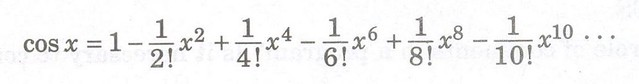CBSE Sample Question Papers for Class 11 Computer Science Set D with Answers are now available for download in PDF format. CBSE Sample Paper are provided below with answers as per the guidelines of CBSE board marking scheme.

Central Board of Secondary Education Board Exam Sample Question Paper for Class 11 are given here. CBSE Class 11 Computer Science Sample Paper gives an idea of question paper pattern and marking scheme.

## CBSE Sample Paper for Class 11 Computer Science (Solved) – Set D

CBSE Sample Paper for Class 11 Computer Science and its solutions are strictly based on the CBSE Board latest syllabus and guidelines. Set D of Solved CBSE Sample Paper for Class 11 Computer Science is given below with its solutions.

## Sample Question Paper

(a) Write one advantage of using Proprietary Software over Open Source Software (OSS). (1 mark)
(b) Define Booting process. (1 mark)
(c) Write two functions of Operating System (2 marks)
(d) Write two characteristics of CISC. (2 marks)

(a) Expand BOSS. (1 mark)
(b) What is the role of comments in a program? Is it necessary to comment every line of code? (2 marks)
(c) Write a short note on Logical Errors with an example. (2 marks)
(d) Convert the following:
(ii) (723)8 to Decimal
(iii) (10101011)2 to BCD (3 marks)

(a) What do you mean by Keyword? (1 mark)
(b) Explain rint() and abs() functions math module with an example. (2 marks)
(c) What is the difference between lower() and islower() ? Give an example illustrating their usage. (2 marks)
(d) Write an algorithm to check whether a person is a tax payer or not. The income of person being the input. (2 marks)
(e) Write various List operations in Python with an example of each. (4 marks)

(a) In reference to Application Software, what do you mean by Inventory Management System? (2 marks)
(b) Write short note on UNIX operating System. (2 marks)
(c) Write a short note on Script mode of Python with an example. (3 marks)
(d) Write some guidelines for effective problem solving. (3 marks)
(e) Draw a flowchart to calculate factorial of a number. (3 marks)
(f) Refer the following code for answering the questions below:
a = [1, 2, 3]
b=[4, 5, 6]
c= a+b
d=c[0:3]
e=d
a=10
c  =20
e = 30
What will be the output of following statements?
(i) print a
(ii) print b
(iii) print d
(iv) print c[:] (4 Marks)

(a) Write a Program in Python to convert °C to K. (2 Marks)
(b) What do you mean by Latency? Should it be less or more for ideal system? (2 Marks)
(c) Given the following code, write down what would be printed as output?
a=3
b=1
for i in range(a) :
for j in range(b) :
print “hello” * i + “oswal” * j (3 Marks)
(d) Write a python program to calculate the cosine series for n terms: (4 marks)(a) What is a function? (1 mark)
(b) Given the following code, there is a single error on each line. Identify the error
» b = like to say + ‘hi’
»c!= b
»println b++c (3 marks)
(c) Given the following python code, what values are printed as output?
def func(B) :
if B>7
print 1
elif B<6
print 0
A=3
afunc(A)
afunc(A+1)
print A (3 marks)
(d) Write a short note on decision statements in Python. (3 marks)